# marcomusy / vtkplotter-examplesFetching latest commit…
Cannot retrieve the latest commit at this time.
Type Name Latest commit message Commit time
..
Failed to load latest commit information.noplotREADME.mdascalarbar.pyawefem.pycalc_surface_area.pycollisions.pycurl2d.pydemo_auto-adaptive-poisson.pydemo_cahn-hilliard.ipynbdemo_cahn-hilliard.pydemo_eigenvalue.pydemo_submesh.ipynbdemo_submesh.pyelasticbeam.ipynbelasticbeam.pyelastodynamics.pyex01_show-mesh.pyex02_tetralize-mesh.pyex03_poisson.ipynbex03_poisson.pyex04_mixed-poisson.ipynbex04_mixed-poisson.pyex05_non-matching-meshes.pyex06_elasticity1.pyex06_elasticity2.ipynbex06_elasticity2.pyex07_stokes-iterative.ipynbex07_stokes-iterative.pyft02_poisson_membrane.ipynbft02_poisson_membrane.pyft04_heat_gaussian.pyft07_navier_stokes_channel.pyft08_navier_stokes_cylinder.pyft09_reaction_system.pyheatconv.pymagnetostatics.ipynbmagnetostatics.pymarkmesh.ipynbmarkmesh.pymeshEditor.pynavier-stokes_lshape.pynodal_u.pypi_estimate.pypointLoad.pyrun_all.shscalemesh.pysimple1Dplot.pystokes.ipynbstokes.pystokes2.pysubmesh_boundary.ipynbsubmesh_boundary.pyturing_2d.pyturing_3d.pyturing_pattern.pywavy_1d.py

# FEniCS/Dolfin examples

In this directory you will find a bunch of examples of to visualize meshes in conjunction with FEniCS/Dolfin package. It emulates the functionality of the `plot()` command of matplotlib. To gain more control on the property of the shown objects see the analogous examples in noplot.

To run the examples:

```git clone https://github.com/marcomusy/vtkplotter-examples.git
cd vtkplotter/examples/other/dolfin
python example.py  # on mac OSX try 'pythonw' instead```

(click thumbnail image to get to the python script)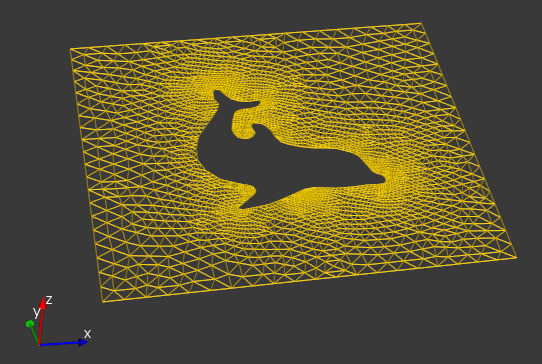`ex01_show-mesh.py`
Show dolfin meshes in different ways.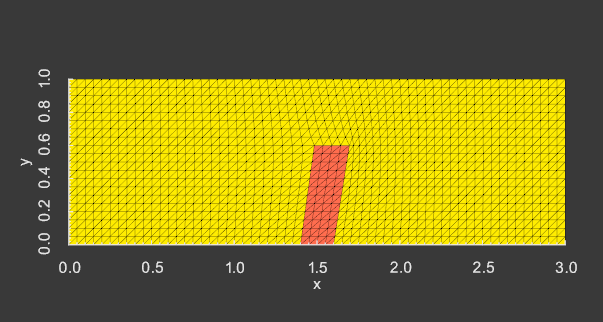`demo_submesh.py`
How to extract matching sub meshes from a common mesh.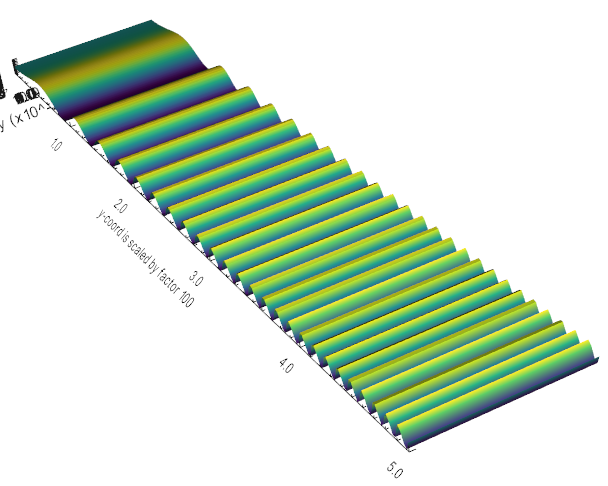`scalemesh.py`
Scale a 2D mesh asymmetrically in one coordinate and elevate it along the z-axis with the solution.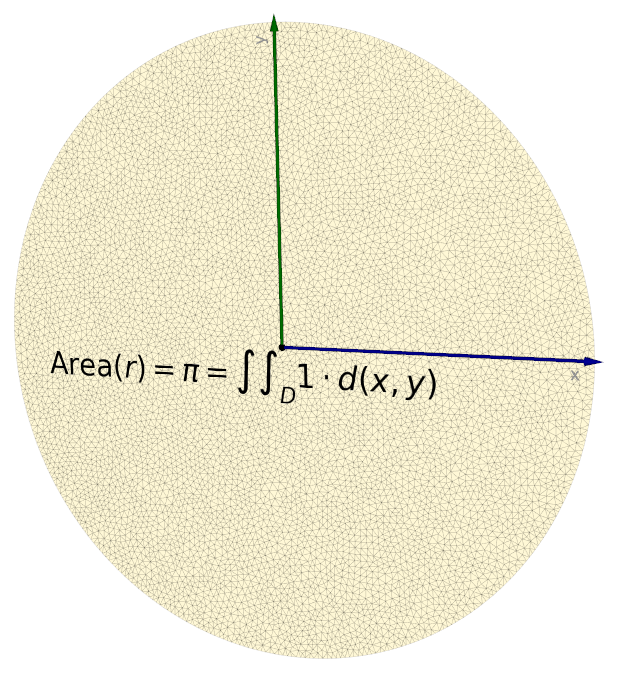`pi_estimate.py`
Estimate pi by integrating a circle surface. Latex formulas can be added to the renderer directly.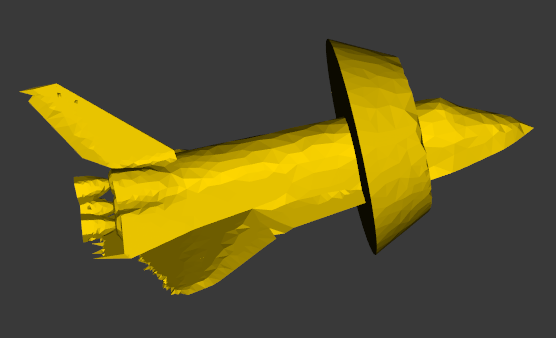`ex02_tetralize-mesh.py`
Tetrahedral meshes generation with package mshr.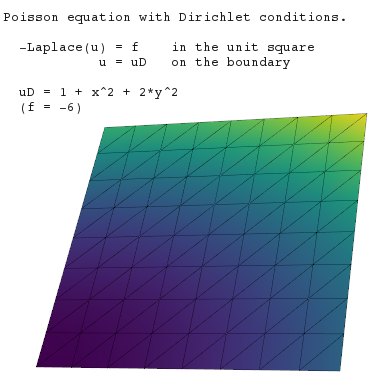`ex03_poisson.py`
Solving Poisson equation with Dirichlet conditions.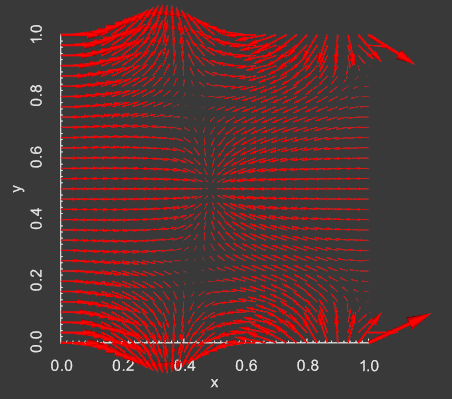`ex04_mixed-poisson.py`
Solving Poisson equation using a mixed (two-field) formulation.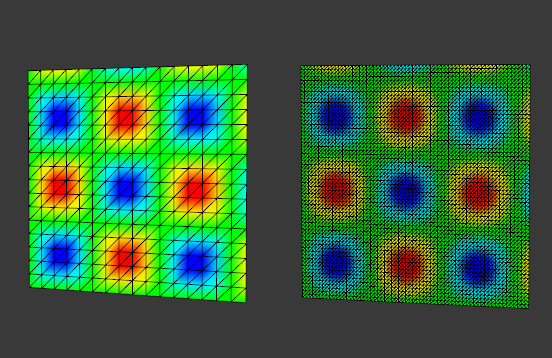`ex05_non-matching-meshes.py`
Interpolate functions between finite element spaces on non-matching meshes.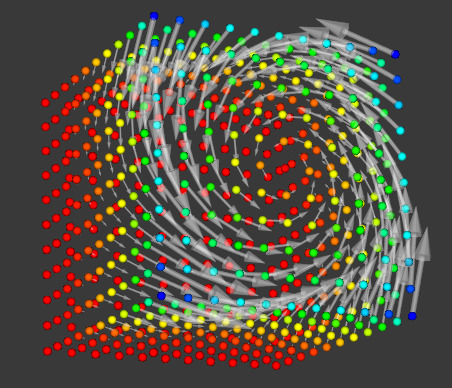`ex06_elasticity1.py`
Solving an elasticity problem. Show mesh displacements with arrows.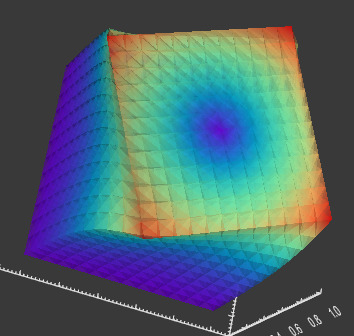`ex06_elasticity2.py`
Solving an elasticity problem. Use scalars and vectors to colorize mesh displacements with different color maps.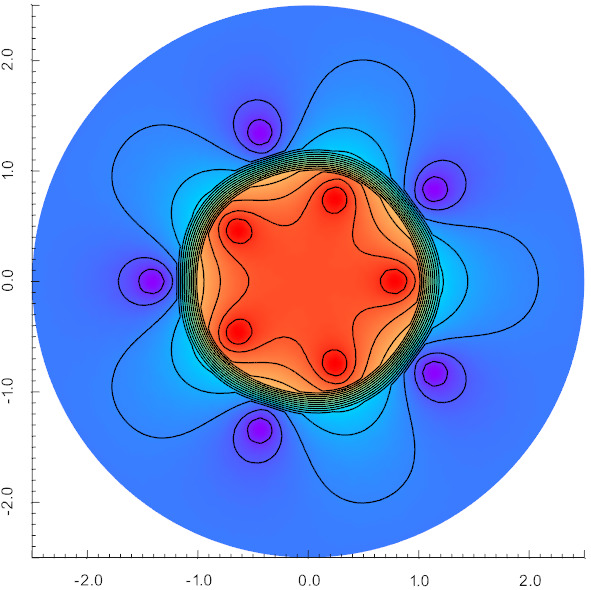`magnetostatics.py`
Compute the magnetic field B in an iron cylinder, the copper wires, and the surrounding vacuum. Isolines of Az are also shown.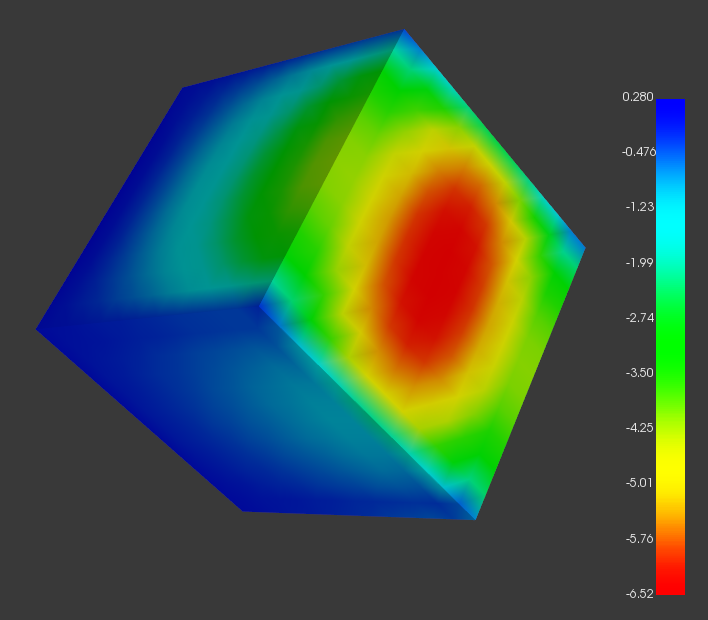`ex07_stokes-iterative.py`
Stokes equations with an iterative solver.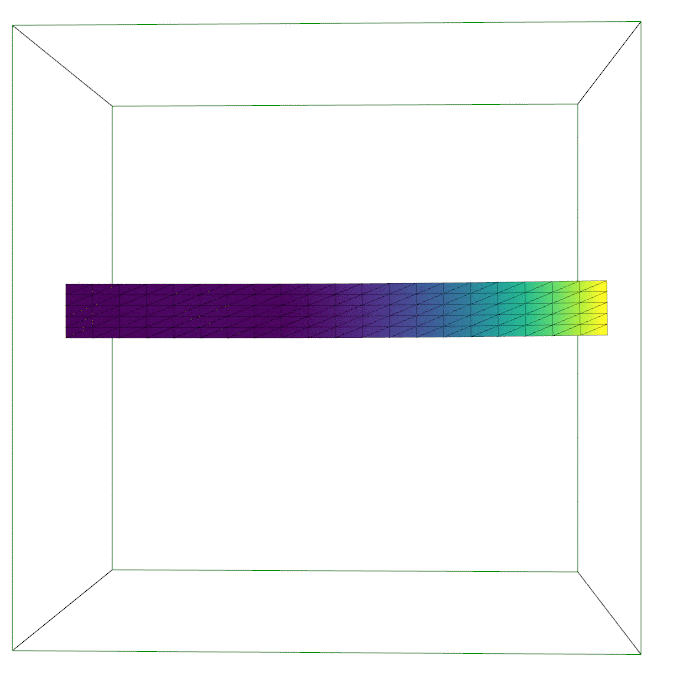`elastodynamics.py`
Perform time integration of transient elastodynamics using the generalized-alpha method.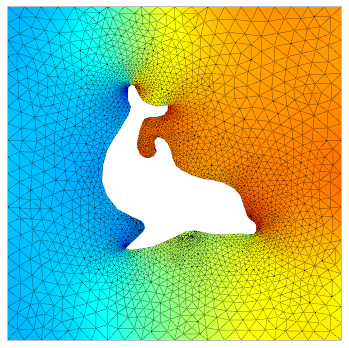`stokes.py`
Solve 2D navier-stokes equations with boundary conditions.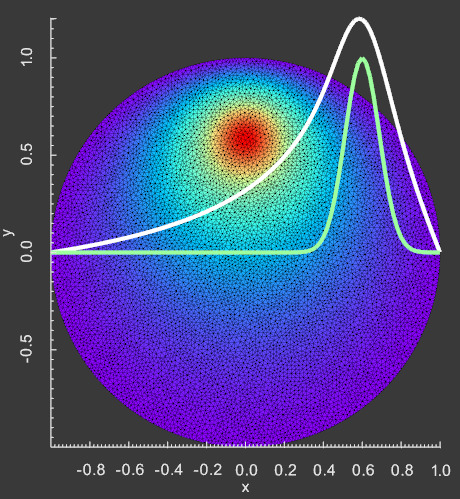`ft02_poisson_membrane.py`
Deflection of a membrane by a gaussian load.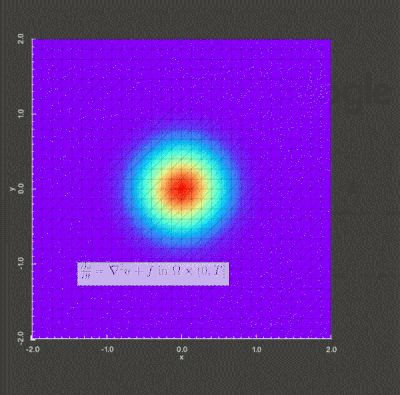`ft04_heat_gaussian.py`
Diffusion of a Gaussian hill on a square domain.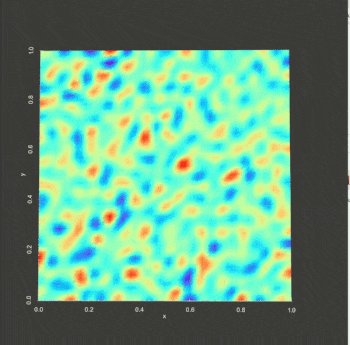`demo_cahn-hilliard.py`
Solution of a particular nonlinear time-dependent fourth-order equation, known as the Cahn-Hilliard equation.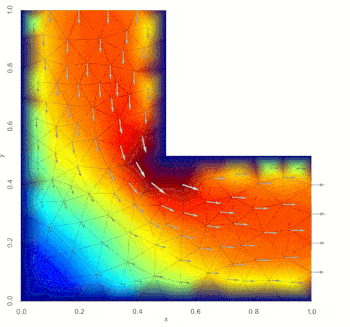`navier-stokes_lshape.py`
Solve the incompressible Navier-Stokes equations on an L-shaped domain using Chorin's splitting method.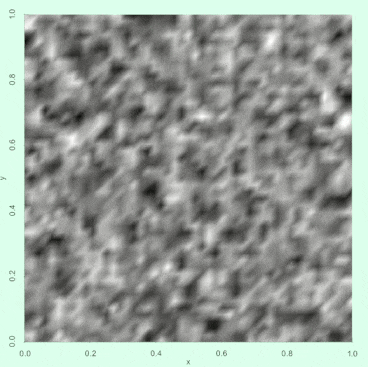`turing_pattern.py`
Solve a reaction-diffusion problem on a 2D domain.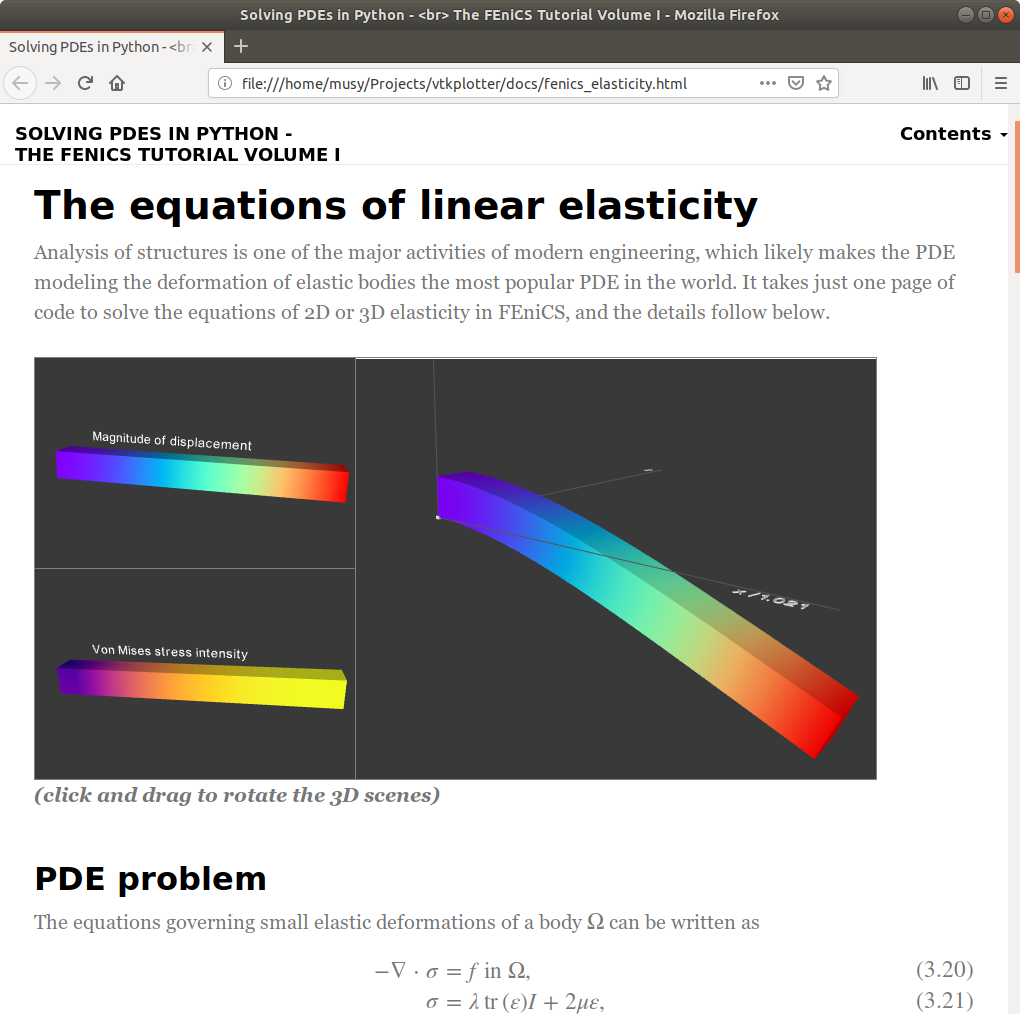`elasticbeam.py`
A clamped beam deformed under its own weight. The whole 3D scene is exported and visualized on a web page.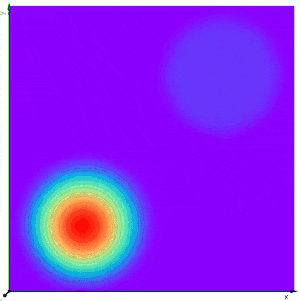`heatconv.py`
Heat equation in moving media. (by J. Blechta).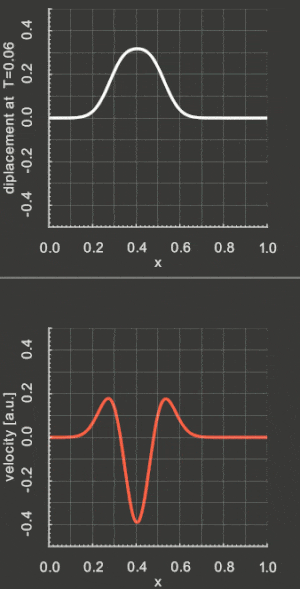`wavy_1d.py`
1-dimensional time integration of the wave equation with the Crank-Nicolson method.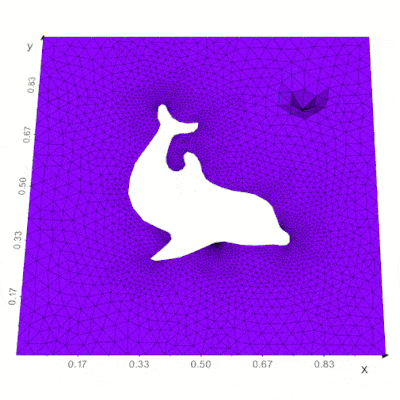`awefem.py`
Solve the constant velocity scalar wave equation in arbitrary number of dimensions.
Original script by C. da Costa.
You can’t perform that action at this time.